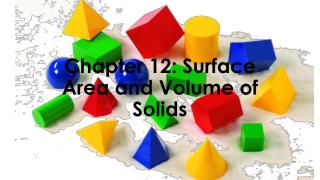# Chapter 12: Surface Area and Volume of Solids - PowerPoint PPT PresentationDownload PresentationChapter 12: Surface Area and Volume of Solids

Chapter 12: Surface Area and Volume of SolidsDownload Presentation## Chapter 12: Surface Area and Volume of Solids

- - - - - - - - - - - - - - - - - - - - - - - - - - - E N D - - - - - - - - - - - - - - - - - - - - - - - - - - -
##### Presentation Transcript

1. Chapter 12: Surface Area and Volume of Solids

2. Polyhedron • A solid that is bounded by polygons, called faces, that enclose a single region of space. • Plural is polyhedral or polyhedrons

3. Face • One of the flat surfaces that make a polyhedron.

4. Edge • A line segment formed by the intersection of two faces of a polyhedron.

5. Vertex • A point where three or more edges of a polyhedron meet.

6. Base • One of two congruent faces of a polyhedron

7. Regular Polyhedron • A convex polyhedron in which all of the faces are congruent regular polygons.

8. Convex Polyhedron • A polyhedron is convex if any two points on its surface can be connected by a segment that lies entirely inside or on the polyhedron. If this segment goes outside the polyhedron, then the polyhedron is nonconvex or concave. convex concave

9. Platonic Solids • A convex polyhedron where every face is an identical regular polygon

10. Tetrahedron • A polyhedron with four faces

11. Cube • A polyhedron with six congruent square faces

12. Octahedron • A polyhedron with eight faces

13. Dodecahedron • A polyhedron with twelve faces

14. Icosahedron • A polyhedron with twenty faces

15. Cross Section • The intersection of a plane and a solid

16. Prism • A polyhedron with two congruent faces called bases that lie in parallel planes.

17. Lateral Faces • The faces of a prism that are parallelograms formed by connecting the corresponding vertices of the bases of the prism

18. Lateral Edges • The segments connecting the corresponding vertices of the bases of a prism

19. Surface Area • The sum of the areas of the faces of a polyhedron or other solid

20. Lateral Area • The sum of the areas of the lateral faces of a polyhedron or other solid with one or two bases.

21. Net • The two-dimensional representation of the faces of a polyhedron

22. Right Prism • A prism in which each lateral edge is perpendicular to both bases

23. Stop for today.

24. Oblique Prism • A prism with lateral edges that are NOT perpendicular to the bases

25. Cylinder • A solid with congruent circular bases that lie in parallel planes

26. Right Cylinder • A cylinder in which the segment joining the centers of the bases is perpendicular to both bases

27. Pyramid • A polyhedron in which the base is a polygon and the lateral faces are triangles with a common vertex

28. Vertex of a Pyramid • The common vertex of the triangles which make up the lateral faces of a pyramid.

29. Regular Pyramid • A pyramid that has a regular polygon for a base and in which the segment joining the vertex and the center of the base is perpendicular to the base

30. Slant Height • The height of a lateral face of the regular pyramid

31. Cone • A solid that has one circular base and a vertex that is not in the same plane as the base

32. Vertex of a Cone • The vertex that does not lie in the same plane as the base of a cone

33. Right Cone • A cone in which the segment joining the vertex and the center of the base is perpendicular to the base. The slant height is the distance between the vertex and a point on the base edge.

34. Lateral Surface • Consists of all segments that connect the vertex with points on the edge of the base.

35. Volume • The number of cubic units contained in the interior of a solid

36. Sphere • The set of all points in space equidistant from a given point called the center of the sphere

37. Center • The center of a polygon’s circumscribed circle

38. Radius • A segment whose endpoints are the center of the circle and a point on the circle. The distance from the center of a circle to any point on the circle. • Plural is radii

39. Chord • A segment whose endpoints are on a sphere

40. Diameter • A chord that contains the center of a sphere

41. Great Circle • The intersection of a sphere and a plane that contains the center of the sphere

42. Hemisphere • Half of a sphere, formed when a great circle separates into two congruent halves

43. Similar Solids • Two solids of the same type with equal ratios of corresponding linear measures, such as heights or radii